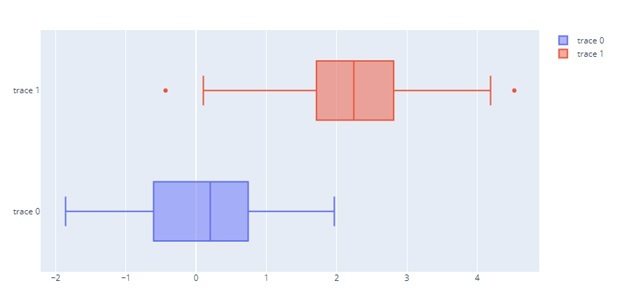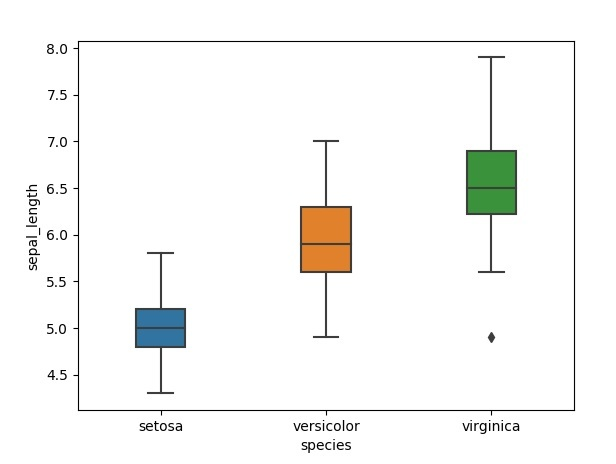# How is violinplot() different from boxplot()?

## What is a violin plot?

A violin plot is a type of statistical chart similar to a box plot but with a rotated kernel density plot on each side. The name "violin plot" comes from the fact that the chart's shape looks similar to a violin's shape.

A violin plot is used to visualise a dataset's distribution and shows the data's probability density at different values. The violin plot displays the data distribution of the sample, with the thickest part showing where the values are most concentrated. In contrast, the skinnier part shows where the values are less concentrated.

## What is a Box plot?

The distribution of a numerical variable for one or more groups is shown in a boxplot. Although it hides the individual dataset data points, it enables easy access to the median, quartiles, and outliers. The Seaborn library's boxplot function makes it easy to create boxplots in Python.

Box plots can only represent so much information, but they are considerably easier to understand, especially when comparing different groups. Although more difficult to understand and visually noisier than density curves, density curves are all about showing distributional information. However, when integrated into a violin plot, the two enhance one another to produce the greatest results possible.

The boxplot() method requires the following syntax −

matplotlib.pyplot.boxplot(data, notch, vert, patch_artist, widths)

Violinplot

Boxplot

Here are the key elements of a violin plot and how to interpret them −

The violin shape − The violin shape is a smoothed version of the histogram of the data, and it shows the probability density of the data at different values. The widest part of the violin represents the highest density of data points. The skinnier parts of the violin represent a lower density of data points.

The box inside the violin − The box inside the violin plot represents the quartiles of the data set. The top and bottom of the box are the upper and lower quartiles, respectively. The line in the middle of the box is the median of the data. The box also shows any outliers as individual points outside the whiskers.

The "stick" inside the violin (if present) − The stick inside the violin represents the raw data points. It gives a sense of the actual distribution of the data points.

The width of the violin − the width of the violin represents the sample size. Wider violin indicates a larger sample size and vice versa.

A box plot is often created using the matplotlib library's boxplot() function.

The Box Plot produces random data via the numpy.random.normal() method. The mean, standard deviation, and desired number of values serve as its arguments.

The data values for the ax.boxplot() function can be an array of arrays in Numpy, a list of arrays in Python, or a tuple of arrays.

Violin charts with Seaborn

A Python package called Seaborn makes it simple to create superior charts. Due to its violin function, it is perfectly suited to creating density charts. You may follow the charts below as they walk you through using it, from a very straightforward violin plot to something much more specialized.

Best practices for using a violin plot

Consider the order of groups.

It is possible to alter the order in which the groups are plotted in a violin plot when there is no intrinsic ordering, which can help you learn more about the data. For instance, sorting groups according to their median value clarify their order.

Vertical vs. horizontal violin plot

A violin plot can be vertically or horizontal. The main difference between the two is the orientation of the plot.

A vertical violin plot is a more common type, and it's plotted with the x-axis as the variable being measured and the y-axis as the frequency or probability density of the data. The violin shape is plotted vertically, with the widest part of the violin representing the highest density of data points.

On the other hand, a horizontal violin plot is plotted with the y-axis as the variable that is being measured and the x-axis as the frequency or probability density. The violin shape is plotted horizontally, with the widest part of the violin representing the highest density of data points.

The choice of plotting a violin plot as vertical or horizontal depends on your specific use case and the data you are working with. In general, the vertical violin plot is more common and the one that is used more frequently, but in some cases, the horizontal violin plot can be more useful to show the comparison.

## Basic Horizontal Box Plot

import plotly.graph_objects as go

import numpy as np

x0 = np.random.randn(50)
x1 = np.random.randn(50) + 2 # shift mean

fig = go.Figure()
# Use x instead of y argument for horizontal plot

fig.show()## boxplot size

The size of the individual boxplots can be altered with the width parameter. The default width is 1, so anything less of that creates a smaller width for the boxes.

import seaborn as sns
import matplotlib.pyplot as plt
sns.boxplot( x=df["species"], y=df["sepal_length"], width=0.3);
plt.show()## Conclusion

Due to the added difficulty of configuring the kernel and bandwidth, violin charts are less prevalent than alternative plots like the box plot. Additionally, it might be aesthetically distracting, especially when a chart type is superimposed. Consider using a simpler and more direct representation, such as the box plot, if you need to come up with a chart to present findings to a group unfamiliar with the violin plot.

A box plot is an underutilized technique that may condense a lot of data-related information into a single visualization. Box plots may be a fantastic addition to histograms when undertaking exploratory data analysis (EDA). One of the first Python visualization tools, Matplotlib offers a large selection of graphs and charts for better analysis.

Updated on: 05-May-2023

181 Views